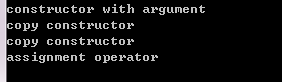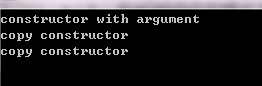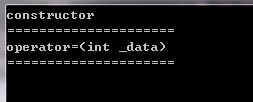2018-05-05 16:53:47 ai_shuyingzhixia 阅读数 341
• ###### 《C语言基础》 之 第3章 运算符

课程内容：表达式、赋值运算符1、赋值预算符2、关系运算符1、关系运算符2、逻辑运算符1 、逻辑运算符2、逻辑运算符3、sizeof运算符1 之 内存结构、sizeof运算符2 之 基本类型回顾 、sizeof运算符3 之 sizeof的使用、逗号运算符 与 符号优先级表

1786 人正在学习 去看看 张先凤

``````#include <iostream>
#include <vector>
#include <algorithm>
#include <stdlib.h>
#include <string.h>
#include <stdio.h>
using namespace std;

class Str{
private:
char *str;
char length;
public:
Str():str(NULL), length(0){}
Str(const Str &s){
length = s.length;
str = (char*)malloc((length + 1)*sizeof(char));
strcpy(str, s.str);
}
Str(char *s, int l){
str = (char*)malloc((l+1)*sizeof(char));
strcpy(str, s);
length = l;
}
int size(){ return length;  }
Str& operator+(const Str &s){
char *ss = (char*)malloc((s.length + length+1)*sizeof(char));
//length = s.length + length;
strcpy(ss, str);
strcat(ss, s.str);
//free(str);
//return Str(ss, s.length + length);
str = ss;
return *this;
}
Str& operator+=(const Str &s){
char *ss = (char*)malloc((s.length + length + 1)*sizeof(char));
length = s.length + length;
strcpy(ss, str);
free(str);
strcat(ss, s.str);
str = ss;
return *this;
}
int operator<=(const Str &s){
if(strcmp(s.str, str) >= 0 )
return 1;
else
return 0;
}
Str& operator=(const Str &s){
if (this == &s) return *this;
length = s.length;
char *ss = (char*)malloc((length + 1)*sizeof(char));
free(str);
strcpy(ss, s.str);
str = ss;
return *this;
}

bool operator==(const Str &s){
if (s.length != length) return false;
else return strcmp(s.str, str) == 0;
}

friend ostream &operator<<(ostream &out, Str &ss){
out << ss.str;
return out;
}
~Str()
{
free(str);
}
};

int main()
{
char s, s2;
strcpy(s, "this is my string");
strcpy(s2, "this is my house");
Str str(s, strlen(s));
Str str2(s2, strlen(s2));
cout << "(str):\t" << str << endl;
cout << "(str2):\t" << str2 << endl;
cout << "(str < str2):\t" << (str<=str2) <<endl;
cout << "sum(str + str2):\t" << str+str2 << endl;
cout << "(str):\t" << str << endl;
cout << "(str2):\t" << str2 << endl;
str += str2;
cout << "excute (str += str2)  str = \t" << str << endl;
cout << "(str2):\t" << str2 << endl;
str = str2;
cout << "excute (str = str2)  str = \t" << str << endl;
cout << "(str2):\t" << str2 << endl;
Str str4 = str2;
cout << "excute (str4 = str2)  str2 = \t" << str2 << endl;
cout << "(str4):\t" << str4 << endl;
cout << "(str4 < str2):\t" << (str4<=str2) <<endl;
return 0;
}``````

c++ 位赋值 相关内容

• ###### 《C语言基础》 之 第3章 运算符

课程内容：表达式、赋值运算符1、赋值预算符2、关系运算符1、关系运算符2、逻辑运算符1 、逻辑运算符2、逻辑运算符3、sizeof运算符1 之 内存结构、sizeof运算符2 之 基本类型回顾 、sizeof运算符3 之 sizeof的使用、逗号运算符 与 符号优先级表

1786 人正在学习 去看看 张先凤

赋值运算符的重载函数基本可以确定为两种写法，一种是初级程序员的写法，另外一种是高级程序员的写法，下面将对赋值运算符的重载函数的两种形式进行解析。

1、初级程序员的写法

```Test& operator=(const Test& src)
{
if(this == &src)
{
return *this;
}
//防止内存丢失和浅拷贝
delete []_ptr;
//此处存在问题
_ptr = new char[strlen(src._ptr)+1];
strcpy_s(_ptr, strlen(src._ptr)+1, src._ptr);
return *this;
}```

2、高级程序员的写法

```Test& operator=(const Test& src)
{
if (this != &src)
{
Test tmp(src);
char *ptmp = tmp._ptr;
tmp._ptr = _ptr;
_ptr = ptmp;
}
return *this;
}```
此代码则很好的解决了上边的问题，在上边的代码片中，我先创建了一个tmp的Test类的临时对象，然后通过ptmp指针将tmp._ptr和(*this)._ptr的指向交换，这样当整个函数指向完后局部对象自动析构，这样就能析构调用operator=()函数的对象的原有的内存，不会产生内存丢失，同时解决了异常安全性的问题。Test的拷贝构造函数里调用new分配内存，如果由于内存不足抛出异常，则还没有修改实例((*this)._ptr)的状态,因此实例的状态还是有效的。

另外，关于赋值运算符的重载函数的返回值为什么是 Test&、形参为什么要加const、形参为什么用引用？这些问题就不再过多陈述，有问题可参考：
http://www.cnblogs.com/zpcdbky/p/5027481.html

c++ 位赋值 相关内容

2019-10-19 11:26:15 qq_29819413 阅读数 31
• ###### 《C语言基础》 之 第3章 运算符

课程内容：表达式、赋值运算符1、赋值预算符2、关系运算符1、关系运算符2、逻辑运算符1 、逻辑运算符2、逻辑运算符3、sizeof运算符1 之 内存结构、sizeof运算符2 之 基本类型回顾 、sizeof运算符3 之 sizeof的使用、逗号运算符 与 符号优先级表

1786 人正在学习 去看看 张先凤

# 代码1，其中给出了自定义的赋值重载函数：

``````class test
{
public:
test()																				// 默认构造函数
{
cout << "constructor with argument\n";
}
~test()																			   // 析构函数
{
}
test(test& t)																		// 复制构造函数
{
cout << "copy constructor\n";
}
test&operator=(const test&e)											// 等号重载函数（赋值重载函数）
{
cout << "assignment operator\n";
return *this;
}
};
int _tmain(int argc, _TCHAR* argv[])
{
test ort;
test a(ort);
test b = ort ;
a = b;
return 0;
}
``````## 要点1：区分两种形式:

``````test b = ort ;  《==》 test b（ort）；   区别于 test b； b=sort；
``````## 要点二：test&operator=(const test&e) 为什么这么写

C++中a = b的含义是在是将b作为operator =(&… )的括号内形参，返回到a上的过程。

### 用const修饰：

①我们不希望在这个函数中对用来进行赋值的“原版”做任何修改。
②加上const，对于const的和非const的实参，函数就能接受；如果不加，就只能接受非const的实参。

### 采用双引用：

①这样在函数返回时避免一次拷贝（若是值传递，函数运行完要把临时变量再付给a），提高了效率。
②更重要的，这样可以实现连续赋值，即类似a=b=c这样。如果不是返回引用而是返回值类型，那么，执行a=b时，调用赋值运算符重载函数，在函数返回时，由于返回的是值类型，所以要对return后边的“东西”进行一次拷贝，得到一个未命名的副本（有些资料上称之为“匿名对象”），然后将这个副本返回，而这个副本是右值，所以，执行a=b后，得到的是一个右值，再执行=c就会出错。

# 代码2，构造函数和赋值重载函数的调用区别

``````#include<iostream>
#include<string>
using namespace std;

class Data
{
private:
int data;
public:
Data() {};
Data(int _data)
:data(_data)																							// 构造函数，形参是个整形
{
cout << "constructor" << endl;
}
Data& operator=(const int _data)															// 赋值重载函数，形参是一个常整形
{
cout << "operator=(int _data)" << endl;
data = _data;
return *this;
}
};

int main()
{
Data data1(1);
Data data2,data3;
cout << "=====================" << endl;
data2 = 1;
cout << "=====================" << endl;
data3 = data2;
return 0;
}
``````

data2 =1；会调用赋值重载函数①如果匹配的构造函数和赋值运算符重载函数同时存在（代码2），会调用赋值运算符重载函数。
②如果只有匹配的构造函数存在，就会调用这个构造函数。

c++ 位赋值 相关内容

2016-03-30 13:57:58 Sico2Sico 阅读数 380
• ###### 《C语言基础》 之 第3章 运算符

课程内容：表达式、赋值运算符1、赋值预算符2、关系运算符1、关系运算符2、逻辑运算符1 、逻辑运算符2、逻辑运算符3、sizeof运算符1 之 内存结构、sizeof运算符2 之 基本类型回顾 、sizeof运算符3 之 sizeof的使用、逗号运算符 与 符号优先级表

1786 人正在学习 去看看 张先凤

1移动赋值运算符.cpp

``````#include<iostream>
#include<string.h>
using namespace std;

class my_string{
char* p=nullptr;
public:
my_string(){}
my_string(const char* s){
if(s){
p = new char[strlen(s)+1];
strcpy(p,s);
}
}
my_string( const my_string& ms){
cout<<"copy construction"<<endl;
if(ms.p){
if(p)delete []p;
p = new char[ms.size()+1];
strcpy(p,ms.p);
}
}
~my_string(){
if(p)delete [] p;
}
my_string(my_string && s)
{
cout<<"move construction"<<endl;
p = s.p;
s.p =nullptr;
}
friend ostream &operator<<(ostream&cout,my_string s);
char & operator[](int pos)
{
return p[pos];
}
int size()const{return strlen(p);}
my_string operator+(my_string &s)
{
my_string tmp;
tmp.p= new char[this->size()+s.size()+1];
strcpy(tmp.p,this->p);
strcat(tmp.p,s.p);
return tmp;
}
my_string operator+(const char* s)
{
my_string tmp;
tmp.p= new char[this->size()+strlen(s)+1];
strcpy(tmp.p,this->p);
strcat(tmp.p,s);
return tmp;
}
my_string &operator=(my_string &&s)
{
cout<<"move assignment=(&&)"<<endl;
p = s.p;
s.p =nullptr;

}
};

ostream &operator<<(ostream&cout,my_string s)
{
cout<<s.p;
return cout;
}

int main()
{
my_string s1="123";
my_string s2="456";
my_string s3;
//不要把一个左值轻易转换为右值使用，除非你确定它在下面不再被使用
//err:  my_string s4(std::move(s1));

//移动赋值运算，提高了运算效率，没有拷贝的过程
s3 =s1+s2;
//(a=b)=c;
int a=1,b=1,c=1;
(a=b)=c;
return 0;
}
``````

c++ 位赋值 相关内容

2014-07-17 12:19:26 CodeAsWind 阅读数 1721
• ###### 《C语言基础》 之 第3章 运算符

课程内容：表达式、赋值运算符1、赋值预算符2、关系运算符1、关系运算符2、逻辑运算符1 、逻辑运算符2、逻辑运算符3、sizeof运算符1 之 内存结构、sizeof运算符2 之 基本类型回顾 、sizeof运算符3 之 sizeof的使用、逗号运算符 与 符号优先级表

1786 人正在学习 去看看 张先凤

Class CMyString

{

Public:

CMyString(char* pData=NULL);

CMyString(const CMyString& str);

~CMyString();

Private:

Char* m_pData;

};

(1)：返回值类型声明为该类型的引用，并且在函数结束前返回实例自身的引用（*this）。

why）只有返回一个引用，才能实现赋值运算符的连续赋值操作。假如返回值为void，并且有三个CMyString对象，那么str1=str2=str3，将不能通过编译。

(2):把传入的参数类型声明为常量的引用。如果传入的参数为实例，那么从形参到实参的过程得调用拷贝构造函数。引发不必要的开销。

(3):释放实例自身的内存，如果在分配新的内存，未释放已有的内存，将造成内存泄露

(4):判断传入的参数和当前的实例是否是同一个实例，若是则不进行赋值操作。如果不进行判断，那么在释放已有内存阶段，同时释放了传入实例的内存，下面将要进行的操作将无效。

CMyString& CMyString：：operator=(const CMyString &str)

{

If(this==&str)

Return *this;

Delete []m_pData;

M_pData=NULL;

M_pData=new char[strlen(str.m_pData)+1];

Strcpy(m_pData,str.m_pData);

Return *this;

}

c++ 位赋值 相关内容